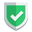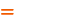我们相信：世界是美好的，你是我也是。平行空间的世界里面，不同版本的生活也在继续...

## 获得鸢尾花训练集

``````import pandas as pd
from sklearn.model_selection import train_test_split

X = iris.data
y = iris.target
X_train, X_test, y_train, y_test = train_test_split(X, y, test_size=0.2, random_state=8)``````

## 逻辑回归模型预测

``````from sklearn.linear_model import LogisticRegression
model = LogisticRegression()
model.fit(X_train, y_train)
predictions = model.predict(X_test)
print(predictions)
print("逻辑回归模型准确度:", model.score(X_test, y_test))``````

``````[0 0 0 2 1 0 0 2 2 1 1 0 1 1 1 2 2 2 2 2 1 0 1 1 1 0 2 0 0 2]

## 可能的报错

``````D:\Program Files\Python368\lib\site-packages\sklearn\linear_model\_logistic.py:765: ConvergenceWarning: lbfgs failed to converge (status=1):
STOP: TOTAL NO. of ITERATIONS REACHED LIMIT.

Increase the number of iterations (max_iter) or scale the data as shown in:
https://scikit-learn.org/stable/modules/preprocessing.html
Please also refer to the documentation for alternative solver options:
https://scikit-learn.org/stable/modules/linear_model.html#logistic-regression
extra_warning_msg=_LOGISTIC_SOLVER_CONVERGENCE_MSG)``````

``model = LogisticRegression(solver='liblinear')``
``model = LogisticRegression(solver='newton-cg')``

## 结束语如果本文对您有帮助，或者节约了您的时间，欢迎打赏瓶饮料，建立下友谊关系。本博客不欢迎：各种镜像采集行为。请尊重原创文章内容，转载请保留作者链接。【福利】 腾讯云最新爆款活动！1核2G云服务器首年50元！【源码】本文代码片段及相关软件，请点此获取更多信息【绝密】秘籍文章入口，仅传授于有缘之人python    sklearn